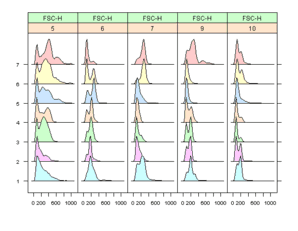Want to share your content on R-bloggers? click here if you have a blog, or here if you don't.

This is the 13th post in a series attempting to recreate the figures in Lattice: Multivariate Data Visualization with R (R code available here) with ggplot2.

Previous parts in this series: Part 1, Part 2, Part 3, Part 4, Part 5, Part 6, Part 7, Part 8, Part 9, Part 10, Part 11, Part 12.

## Chapter 14 – New Trellis Displays

Topics covered:

• Examples of S3 and S4 methods
• Examples of new high level functions

### Figure 14.1

 ```> library(lattice) > library(ggplot2) > library(latticeExtra)```

lattice

 ```> pl <- xyplot(sunspot.year, aspect = "xy", strip = FALSE, + strip.left = TRUE, cut = list(number = 4, overlap = 0.05)) > print(pl)```

ggplot2

 ```> sunspot.g <- function(data, number = 4, overlap = 0.05) { + data <- as.data.frame(data) + data\$id <- if (is.ts(data\$x)) + time(data\$x) + else seq_along(data\$x) + intrv <- as.data.frame(co.intervals(data\$id, number, + overlap)) + x <- sort(unique(data\$id)) + intervals <- ldply(x, function(x) { + t(as.numeric(x < intrv\$V2 & x > intrv\$V1)) + }) + tmp <- melt(cbind(x, intervals), id.var = 1) + tmp <- tmp[tmp\$value > 0, 1:2] + tmp <- rename(tmp, c(x = "id")) + merge(data, tmp) + }```
 ```> pg <- ggplot(sunspot.g(sunspot.year), aes(id, x)) + geom_line() + + facet_wrap(~variable, scales = "free_x", ncol = 1, + as.table = FALSE) + opts(strip.background = theme_blank(), + strip.text.x = theme_blank()) + opts(panel.margin = unit(-0.25, + "lines")) + xlab("Time") > print(pg)```

### Figure 14.2

 `> data(biocAccess, package = "latticeExtra")`

lattice

 ```> ssd <- stl(ts(biocAccess\$counts[1:(24 * 30 * 2)], frequency = 24), + "periodic")```
 ```> pl <- xyplot(ssd, xlab = "Time (Days)") > print(pl)```

ggplot2

 ```> time <- data.frame(data = ts(biocAccess\$counts[1:(24 * + 30 * 2)], frequency = 24)) > time\$id <- as.numeric(time(time\$data)) > time\$data <- as.numeric(time\$data) > time.series <- as.data.frame(ssd\$time.series) > time.series <- cbind(time, time.series) > time.series <- melt(time.series, id.vars = "id")```
 ```> pg <- ggplot(time.series, aes(id, value)) + geom_line() + + facet_grid(variable ~ ., scales = "free_y") + xlab("Time (Days)") > print(pg)```

### Figure 14.3

 ```> library("flowViz") > data(GvHD, package = "flowCore")```

lattice

 ```> pl <- densityplot(Visit ~ `FSC-H` | Patient, data = GvHD) > print(pl)```

ggplot2

 `It should be possible to produce a similar graph in ggplot2, however I was not able to figure out how to extract the relevant data from an object of class "flowSet".`### Figure 14.4

 ```> library("hexbin") > data(NHANES)```

lattice

 ```> pl <- hexbinplot(Hemoglobin ~ TIBC | Sex, data = NHANES, + aspect = 0.8) > print(pl)```

ggplot2

 ```> pg <- ggplot(NHANES, aes(TIBC, Hemoglobin)) + geom_hex() + + facet_grid(~Sex) + opts(aspect.ratio = 0.8) > print(pg)```

### Figure 14.5

 `> data(Chem97, package = "mlmRev")`

lattice

 ```> panel.piechart <- function(x, y, labels = as.character(y), + edges = 200, radius = 0.8, clockwise = FALSE, init.angle = if (clockwise) 90 else 0, + density = NULL, angle = 45, col = superpose.polygon\$col, + border = superpose.polygon\$border, lty = superpose.polygon\$lty, + ...) { + stopifnot(require("gridBase")) + superpose.polygon <- trellis.par.get("superpose.polygon") + opar <- par(no.readonly = TRUE) + on.exit(par(opar)) + if (panel.number() > 1) + par(new = TRUE) + par(fig = gridFIG(), omi = c(0, 0, 0, 0), mai = c(0, + 0, 0, 0)) + pie(as.numeric(x), labels = labels, edges = edges, + radius = radius, clockwise = clockwise, init.angle = init.angle, + angle = angle, density = density, col = col, + border = border, lty = lty) + } > piechart <- function(x, data = NULL, panel = "panel.piechart", + ...) { + ocall <- sys.call(sys.parent()) + ocall[] <- quote(piechart) + ccall <- match.call() + ccall\$data <- data + ccall\$panel <- panel + ccall\$default.scales <- list(draw = FALSE) + ccall[] <- quote(lattice::barchart) + ans <- eval.parent(ccall) + ans\$call <- ocall + ans + } > pl <- piechart(VADeaths, groups = FALSE, xlab = "") > print(pl)```

ggplot2

 ```> pg <- ggplot(as.data.frame.table(VADeaths), aes(x = factor(1), + y = Freq, fill = Var1)) + geom_bar(width = 1) + facet_wrap(~Var2, + scales = "free_y") + coord_polar(theta = "y") > print(pg)```